# Factors MultiplesPage 1

#### WATCH ALL SLIDES

Slide 1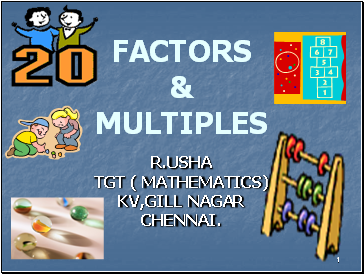1

FACTORS & MULTIPLES

R.USHA

TGT ( MATHEMATICS)

KV,GILL NAGAR

CHENNAI.

Slide 22

## Arrangement of stars

Take 6 stars and arrange

them in different

ways

Slide 3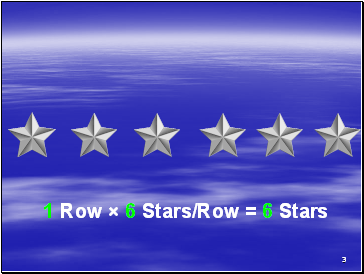3

1 Row × 6 Stars/Row = 6 Stars

Slide 4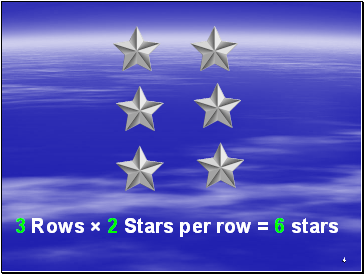4

3 Rows × 2 Stars per row = 6 stars

Slide 5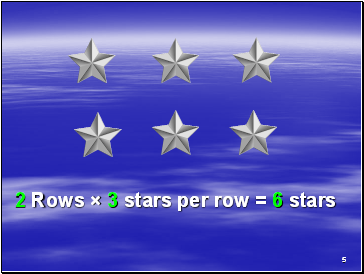5

2 Rows × 3 stars per row = 6 stars

Slide 6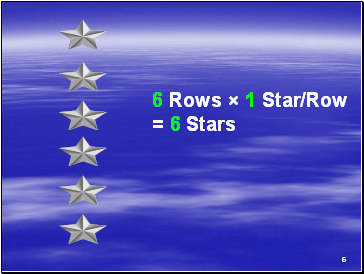6

6 Rows × 1 Star/Row = 6 Stars

Slide 7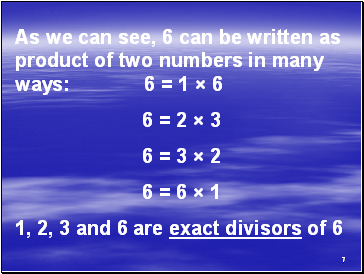7

As we can see, 6 can be written as product of two numbers in many ways: 6 = 1 × 6

6 = 2 × 3

6 = 3 × 2

6 = 6 × 1

1, 2, 3 and 6 are exact divisors of 6

Slide 8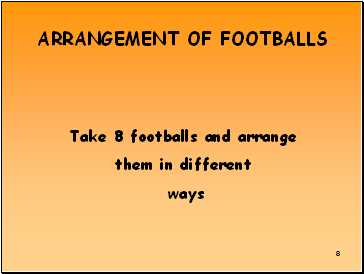8

## Arrangement of footballs

Take 8 footballs and arrange

them in different

ways

Slide 9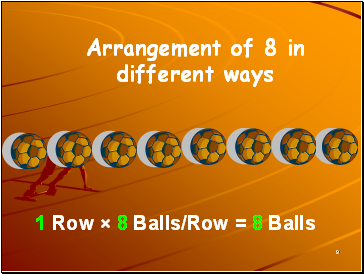9

Arrangement of 8 in different ways

1 Row × 8 Balls/Row = 8 Balls

Slide 10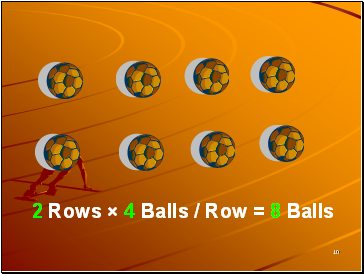10

2 Rows × 4 Balls / Row = 8 Balls

Slide 11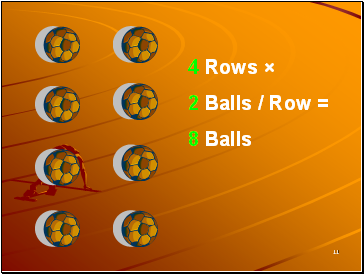11

4 Rows ×

2 Balls / Row =

8 Balls

Slide 12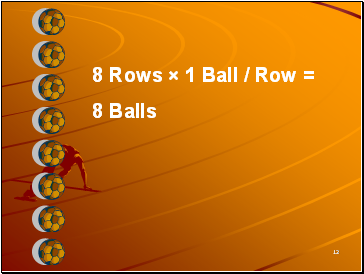12

8 Rows × 1 Ball / Row =

8 Balls

Slide 1313

1, 2, 4 and 8 are exact divisors of 8.

Slide 14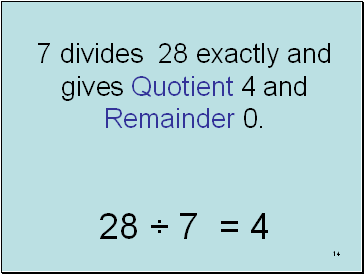14

7 divides 28 exactly and gives Quotient 4 and Remainder 0.

28 ÷ 7 = 4

Slide 15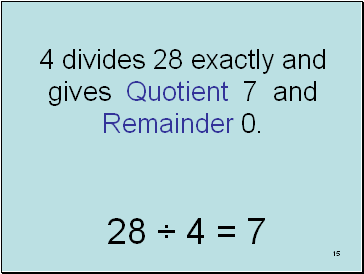15

4 divides 28 exactly and gives Quotient 7 and Remainder 0.

28 ÷ 4 = 7

Slide 16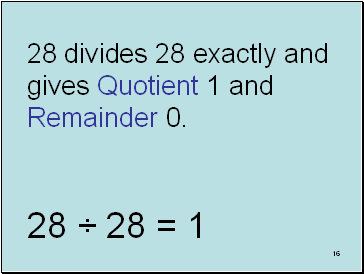16

28 divides 28 exactly and gives Quotient 1 and Remainder 0.

28 ÷ 28 = 1

Slide 17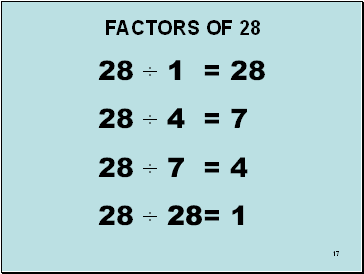17

Go to page:
1  2  3